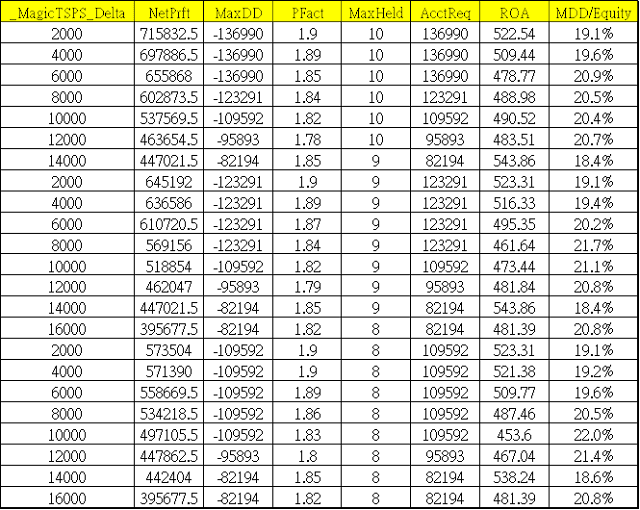## 2016年5月2日 星期一

### 開發商品的交易系統 - 進階篇 

“風險管理是應當被理解的最重要的事情。縮減你的部位是我的第二個建議。不論你認為應該持有多少部位，先砍掉一半。”－Bruce Kovner。

“為了那些在很短的時間內大幅度獲利的機會，你必須最小化損失並且盡可能的保存你的資本。你不能做的事情就是在不合適的時機扔掉自己的資本”－Richard Dennis。PositionSizing = 0.5 * (1 + squareroot(1 + 8 * Equity/Delta))  {近似值}

{系統參數與變數}
Inputs:InitialCapital(100000),Delta(10000),upBand(1),DnBand(8);
Inputs:InCludeOpenPL(false),MaxSize(20);
Var:ContractAmt(0),ExitAmount(0),Equity(0);

{由於帳戶權益金額是決定部位規模變化的基礎，所以加上了 Equity 的計算，同時也放入是否將未平倉盈虧 OpenPositionProfit 值納入考量}

if InCludeOpenPL then Equity = Round((InitialCapital + NetProfit + OpenPositionProfit),0)
else Equity = Round((InitialCapital + NetProfit),0);

{Position Sizing - Fixed Ratio }{固定比率}
If Delta > 0 and Equity/Delta > 0 then ContractAmt = 0.5 * (1 + squareroot(1 + 8 * Equity/Delta));
ContractAmt = MaxList(1, IntPortion(ContractAmt));

if ContractAmt < 1 then ContractAmt = 1 ; {維持最小口數}
if ContractAmt > MaxSize then ContractAmt = MaxSize ; {最大口數限制}

{空手時，當收盤價小於過去N日低點均價時進場作多}
If Marketposition = 0 then begin
If Close < Average(Low,DnBand) then begin
Buy ContractAmt Contracts next bar at market;
ExitAmount = ContractAmt;
end;
end;

{多單持倉，當收盤價大於過去N日高點均價時進場平倉}
If Marketposition = 1 then begin
if Close > Average(High,upBand) then begin
ExitLong ExitAmount Contracts next bar at Market;
end;
end;